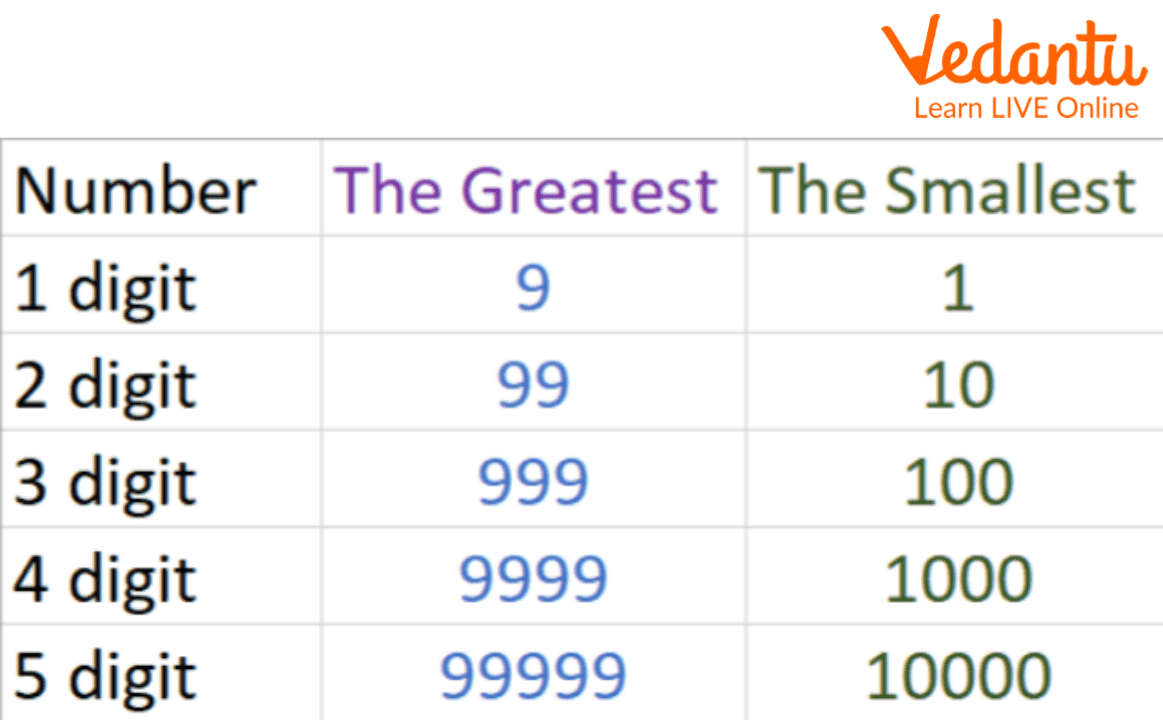Courses
Courses for Kids
Free study material
Free LIVE classes
More

# The Smallest and Greatest Number## What is Greatest Number and the Smallest Number in Maths?

The smallest number in maths is 1. There is no end to numbers in mathematics. In mathematics, 1 is the smallest number and the largest number has no end. Apart from this, there are many other numbers in mathematics such as composite numbers, prime numbers, etc.One-Digit Number: Smallest and Greatest Number

The Smallest 1 digit number is 1 and the Greatest one-digit number is 9. Based on this, we can find whatever number we want to find from the smallest number to the greatest number.Name of the Chapter: The Smallest and Greatest Number

## How to Find the Smallest and the Greatest Number of any Digit?

The smallest 2-digit number will become 10 and the largest number will become 99 To make or write the smallest number we write a zero next to one becomes the smallest 2-digit number so on. To write the smallest number, write two zeros next to one, and then it will become the smallest number of three digits. Similarly, if you want to write the smallest number of 4 digits, 5 digits, 6 digits, and 9 digits, after writing one, write zero so many times that the number of digits becomes.

Like the smallest 8-digit number will be 10000000 as it is written 7 times zero after one.

Similarly, you can write the smallest number of any digit to write that number after writing one so much zero should be made that it becomes that number of digits.

Similarly, the Greatest number of any digit can also be written as the Greatest two-digit number is 99 in this you will see that 9 is written 2 times. That is, you can know that to write the largest number as many times as 9 is written then it will become the largest number of that digit e.g. the largest number of 8 digits will be 99999999, it will be the largest number of 8-digit numbers. Now that I understand how to write the smallest number and the largest number.Smallest and Greatest Numbers Chart

## Solved Questions

1. Which is the smallest number?

Ans: 1 is the smallest number.

1. Which is the smallest whole number?

Ans: 0 is the smallest whole number.

1. What is the largest two-digit natural number(a part of the number system, which includes all positive integers from 1 to infinity)?

Ans: 99 is the largest two-digit number.

1. What is the smallest 3-digit number?

Ans: 100 is the smallest 3-digit number.

1. What is the smallest even number(Such natural numbers which are exactly divisible by 2)?

Ans: 2 is the smallest even number.

### Summary

First of all, we know which is the smallest number of one digit and which is the largest number, which is known, then based on this, we can find the smallest and largest number of any digit. The smallest one-digit number is 1 and the largest one-digit number is 9. Based on this, we can find whatever number we want to find from the smallest number to the greatest number.

## Learning by Doing

Write True or False.

1. The infinity of numbers exists.

2. 99999 is the largest 4-digit number.

3. 9 is the largest single-digit number.

4. 999999999 is the largest 7-digit number.

Last updated date: 30th Sep 2023
Total views: 78k
Views today: 0.78k

## FAQs on The Smallest and Greatest Number

1. What is the smallest negative number?

The smallest negative number is - infinity.

2. What is the largest 6-digit number?

999999 is the largest 6-digit number.

What is the largest two-digit prime number?

97 is the largest two-digit prime number.